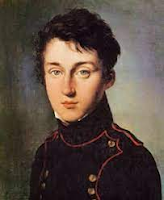# Define Carnot Theorem and Give its Proof.

Carnot theorem states that no heat engine working in a cycle between two constant temperature reservoirs can be more efficient than a reversible engine working between the same reservoirs. In other words it means that all the engines operating between a given constant temperature source and a given constant temperature sink, none, has a higher efficiency than a reversible engine.

Proof : -
Suppose there are two engines EA and Eoperating between the given source at temperature T1 and the given sink at temperature T2.

Let EAbe any irreversible heat engine and EB be any reversible heat engine. We have to prove that efficiency of heat engine EB is more than that of heat engine EA.

Suppose both the heat engines receive same quantity of heat Q from the source at temperature T1. Let WA and WB be the work output from the engines and their corresponding heat rejections be (Q – WA) and (Q – WB) respectively.

Assume that the efficiency of the irreversible engine be more than the reversible engine i.e. ηA > ηB. Hence,
WA / Q > WB / Q
i.e. WA > WB

Now let us couple both the engines and EB is reversed which will act as a heat pump. It receives (Q – WB) from sink and WA from irreversible engine Eand pumps heat Q to the source at temperature T1. The net result is that heat WA – WB is taken from sink and equal amount of work is produce. This violates second law of thermodynamics. Hence the assumption we made that irreversible engine having higher efficiency than the reversible engine is wrong.

Hence it is concluded that reversible engine working between same temperature limits is more efficient than irreversible engine thereby proving Carnot’s theorem.Nicolas Sadi Carnot